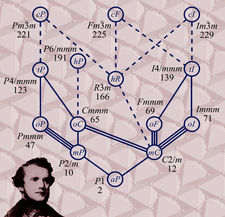issue contentsFOUNDATIONSADVANCES
ISSN: 2053-2733

March 2015 issueCover illustration: Bravais described his complete set of space-filling lattices in 1850, but new insights into the hierarchical relationship between the Bravais lattices described in this issue [Grimmer (2015). Acta Cryst. A71, 143-149; see also Flack (2015). Acta Cryst. A71, 141-142] will give scientists powerful new tools in predicting new structures and understanding phase transitions, such as the magnetic ordering in haematite (shown as a backdrop). Image courtesy of K. M. Ø. Jensen, Columbia University.

scientific commentaries

The revival of the Bravais lattice

Crystallographers need to understand better the Bravais lattice.

research papers

Partial order among the 14 Bravais types of lattices: basics and applications

The partial order among Bravais types of lattices obtained by considering special cases is derived from their space-group symmetry and applied to continuous equi-translation phase transitions.

research papers

The affine and Euclidean normalizers of the subperiodic groups

The affine and Euclidean normalizers of the subperiodic groups are derived and listed.

Generalized Penrose tiling as a quasilattice for decagonal quasicrystal structure analysis

Decorated generalized Penrose tiling is described as a potential quasilattice for models of decagonal quasicrystals. Its advantage over the conventional Penrose tiling is that its long-range order can be continuously changed if the tile decoration is fixed.

A simple approach to estimate isotropic displacement parameters for hydrogen atoms

A simple approach to estimate temperature-dependent isotropic motion of hydrogen atoms is proposed. The model is validated against experimental data.

Group-theoretical analysis of aperiodic tilings from projections of higher-dimensional lattices Bn

A general technique has been introduced for the projection of the hypercubic lattices into two- and three-dimensional subspaces with dihedral and icosahedral residual symmetries, respectively. Eigenvalues and corresponding eigenvectors of the Cartan matrix (Gram matrix) determine the projected subspace and symmetry of the aperiodic tilings.

Mathematical aspects of molecular replacement. III. Properties of space groups preferred by proteins in the Protein Data Bank

In order to characterize molecular-replacement search spaces, the structure of Sohncke groups is examined. It is observed that proteins most often crystallize in Sohncke groups with small torsion subgroups.

Twinning of aragonite – the crystallographic orbit and sectional layer group approach

The mimetic twinning of aragonite is explained by the high degree of pseudo-symmetry of the crystallographic orbits and the action of the twin operation on the structure slices which form the composition surface.

Statistical tests against systematic errors in data sets based on the equality of residual means and variances from control samples: theory and applications

Residuals from artificial and from published data are tested against the hypothesis of being identically distributed. An anharmonic motion model reduces the number of rare events in the lowest resolution range.

On the number of k-faces of primitive parallelohedra

Linear relations between numbers of k-faces of non-principal primitive parallelohedra are obtained from Dehn–Sommerville relations for simple polytopes.

Color groups arising from index-n subgroups of symmetry groups

Given a subgroup H of the symmetry group G of an object, an H-invariant coloring of the object has a color group which is a subgroup of G containing H. A method is described for determining whether this subgroup is G, H or some intermediate subgroup.

Computational analysis of thermal-motion effects on the topological properties of the electron density

Correlations between different local topological properties of the electron density due to nuclear vibrations are analysed via computational statistics.

Structure refinement using precession electron diffraction tomography and dynamical diffraction: theory and implementation

A method for structure refinement from electron diffraction tomography data is introduced.

short communications

Density- and wavefunction-normalized Cartesian spherical harmonics for l ≤ 20

Cartesian real spherical harmonics for l ≤ 20 and the corresponding normalization factors for the deformation density functions with an accuracy to 35 significant figures have been generated using the Wolfram Mathematica software and converted to a Fortran90 code.

obituaries

Hans Wondratschek (1925–2014)

Obituary for Hans Wondratschek.
Follow Acta Cryst. A